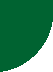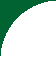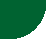FORECASTINGHome Page

PRAXIS

 Forecasting is a management endeavor to foretell how business is going to be next term. It is performed by qualitative and quantitative techniques.   Qualitative techniques are forecasting procedures which utilize judgment and experience to produce estimates of future events.   The Delphi Method, Market Research and Historical Analogy are examples of qualitative forecasting techniques.   Quantitative techniques are forecasting procedures which use past data to establish a pattern that will determine the nature of future estimates. These techniques assume that the past is an indication of what is going to happen in the future.   Several statistical techniques are specifically designed for forecasting, the most common of them being regression analysis.   Regression Analysis   Regression analysis is a statistical technique that provides a mathematical model that better represents a series of observations.   Linear Regression   If the observations at hand are suggesting a linear relationship of the form: Y = A + BX   Where A and B are constants, A is the ordinate value where the straight line representing the above-shown equation intersects the Y axis, B is the slope of the straight line representing the same equation or the ratio Y/X.   Linear regression provides the means of calculating the values of A and B characteristic of the particular observations by resolving the following simultaneous equations:   ΣY = A*n + B * ΣX ΣXY = A*ΣX + B*ΣX2   Where n is equal to number of pairs of observations and, ΣX = sum of periods ΣY = sum of observations To get a full chapter on forecasting, follow the internet link shown below: https://app.box.com/s/vyljgkbpiu0xios84kizMORE MATTER WITH LESS ART